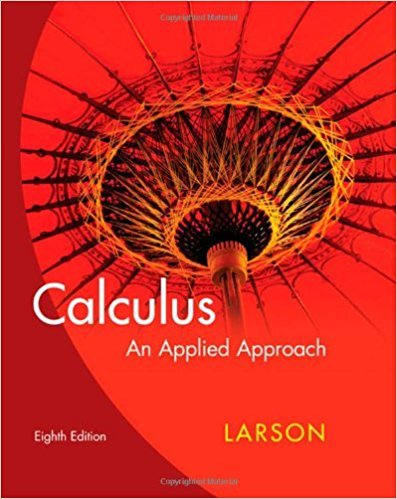×
×

# Solutions for Chapter 7.4: Partial Derivatives## Full solutions for Calculus: An Applied Approach | 8th Edition

ISBN: 9780618958252Solutions for Chapter 7.4: Partial Derivatives

Solutions for Chapter 7.4
4 5 0 333 Reviews
12
4
##### ISBN: 9780618958252

Chapter 7.4: Partial Derivatives includes 70 full step-by-step solutions. Since 70 problems in chapter 7.4: Partial Derivatives have been answered, more than 21824 students have viewed full step-by-step solutions from this chapter. This expansive textbook survival guide covers the following chapters and their solutions. Calculus: An Applied Approach was written by and is associated to the ISBN: 9780618958252. This textbook survival guide was created for the textbook: Calculus: An Applied Approach , edition: 8.

Key Calculus Terms and definitions covered in this textbook
• Aphelion

The farthest point from the Sun in a planet’s orbit

• Arcsine function

See Inverse sine function.

• Bounded

A function is bounded if there are numbers b and B such that b ? ƒ(x) ? B for all x in the domain of f.

• Data

Facts collected for statistical purposes (singular form is datum)

• Discriminant

For the equation ax 2 + bx + c, the expression b2 - 4ac; for the equation Ax2 + Bxy + Cy2 + Dx + Ey + F = 0, the expression B2 - 4AC

• Focal length of a parabola

The directed distance from the vertex to the focus.

• Horizontal asymptote

The line is a horizontal asymptote of the graph of a function ƒ if lim x:- q ƒ(x) = or lim x: q ƒ(x) = b

• Horizontal Line Test

A test for determining whether the inverse of a relation is a function.

• Independent events

Events A and B such that P(A and B) = P(A)P(B)

• Initial point

See Arrow.

• Inverse cosecant function

The function y = csc-1 x

• Negative linear correlation

See Linear correlation.

• One-to-one function

A function in which each element of the range corresponds to exactly one element in the domain

• Origin

The number zero on a number line, or the point where the x- and y-axes cross in the Cartesian coordinate system, or the point where the x-, y-, and z-axes cross in Cartesian three-dimensional space

• Outliers

Data items more than 1.5 times the IQR below the first quartile or above the third quartile.

• Parallelogram representation of vector addition

Geometric representation of vector addition using the parallelogram determined by the position vectors.

• Parametric curve

The graph of parametric equations.

The formula x = -b 2b2 - 4ac2a used to solve ax 2 + bx + c = 0.

• Right triangle

A triangle with a 90° angle.

• Terminal point

See Arrow.

×